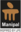Trending ▼   ResFinder# ISC Class XII Prelims 2021 : Computer Science (Madhusthali Vidyapeeth, Deoghar)

5 pages, 32 questions, 0 questions with responses, 0 total responses,20Soumya Roy Sikkim Manipal University (SMU), Gangtok Master of Computer Application Computer Application
+Fave Message
 Home > coolsroy_mdp >   F Also featured on: iscFormatting page ...

MADHUSTHALI VIDYAPEETH F.M 70 Time 3h Date : PRE-BOARD EXAMINATION: 2020-2021 SUBJECT: COMPUTER SCIENCE CLASS- XII (Candidates are allowed additional 15 minutes for only reading the paper. They must NOT start writing during this time.) Answer all questions in Part I (compulsory) and six questions from Part II, choosing two questions from Section A, two from Section B and two from Section C. All working, including rough work, should be done on the same sheet as the rest of the answer. The intended marks fir questions or parts of questions are given in brackets [ ]. PART I (20 Marks) Answer all questions. While answering questions in this part, indicate briefly your working and reasoning, wherever required. Question 1 (a) State the properties of zero in Boolean algebra. (b) Find the complement of the following Boolean expression using De Morgan s law: F(P, Q, R) = P + (Q . R) (c) Find the dual of: (A + 0) . (B + 1) = A (d) State whether the following proposition is a tautology, contradiction or a contingency: F = (P Q) (Q ~P) (e) Study the diagram given below and answer the questions that follow: (i) Name the basic gate which is represented by the diagram. (ii) What will be the value of X when A = 1 and B = 0? Question 2 (a) State the difference between a Binary Tree structure and a Stack. (b) A matrix B is stored in the memory with each element requiring 2 bytes of storage. If the base address at B is 2140, find the address of B when the matrix is stored in Column Major Wise. (c) Convert the following infix notation to prefix form: (X + Y) / (Z * W / V) (d) State the best case and the worst case complexity for bubble sort algorithm. (e) What is the significance of the keyword new in Java? Mention the areas where it is used. Question 3 The following function check() is a part of some class. What will the function check() return when the value of (i) n = 25 and (ii) n = 10. Show the dry run/working. int check(int n){ if(n <= 1) return 1; if(n % 2 == 0) return 1 + check(n / 2); else return 1 + check(n / 2 + 1); } 1Formatting page ...Formatting page ...Formatting page ...Formatting page ...